## Hopalong Fractals

Discovered by Barry Martin from Aston University (UK), presented by A.K. Dewdney presented in "Scientific American" (September, 1986).

A fractal is a geometric structure that displays self-similar patterns. Popular fractals are the Mandelbrot set or the Sierpinski triangle. This experiment generates Hopalong fractals which are generated be repeatedly applying a mathematical rule to produce an infinite sequence of two-dimensional (x, y) points. We always start our sequence in the origin of the plane, i.e., the point (x0, y0) = (0, 0). From there, we apply the mathematical rule to the last point and generate the next one step by step. We call the last produced point (xn, yn) and say that it has been generated after n steps. To get the next point, i.e., (xn+1, yn+1) we use the rule: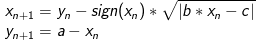where sign() returns the sign (-1, 1 or, 0) of xn and a, b, and c ar free variables that we choose in the beginning and keep fixed for the sequence. The shape of the fractal primarily depends on the choice of these variables. When generating one point after another, we can imagine the function to hop along the trajectory of the fractal (which gives it the name Hopalong).

While the plane consists of infinite many points, the computer has only a finite amount of pixels. Imagine, we lay the pixel grid over the plane and whenever we generate another point, we color the pixel within the point has fallen. Not only do we color each pixel but also count how many points have already fallen into it and to choose a different color according to that count. The pixels hit most are going to be red and the pixels with lesser hits gradually more purple, bluer, greener and at last yellow. Pixels that have not yet been hit are going to remain black.

In the following example, we have chosen a = -11, b = 0.05, c = 0.5, and set the resolution to 10 pixels per unit, i.e., 100 pixels per unit square.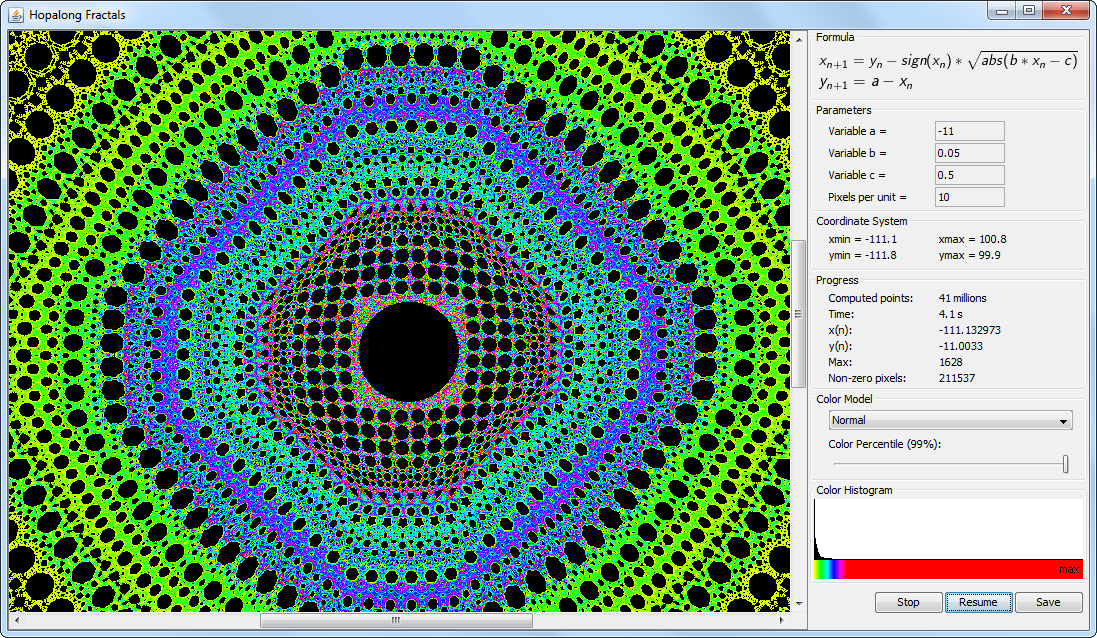a = 1.1b = 1 c = 0 R = 50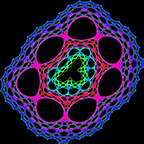a = 2b = 1 c = 0 R = 50a = 7.3b = 1 c = 0 R = 50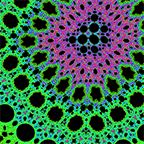a = 5b = 1 c = 20 R = 5a = -11b = 0.05 c = 0.5 R = 20a = 1b = 0.1 c = 20 R = 5a = 1.1b = -0.5 c = 1 R = 5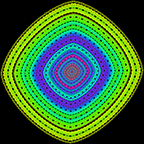a = 1.1b = 0.5 c = 1 R = 5a = 2b = 0.05 c = 4 R = 5